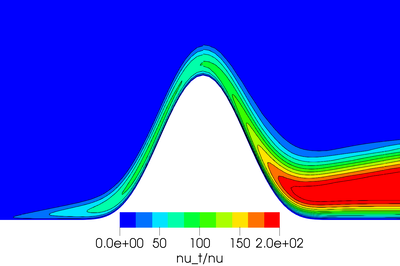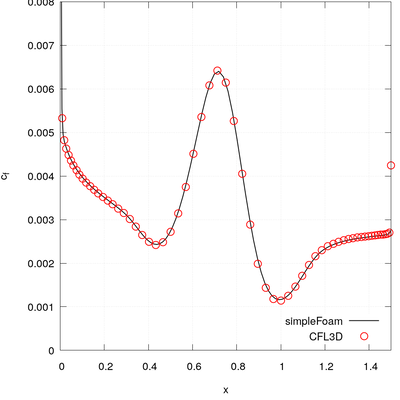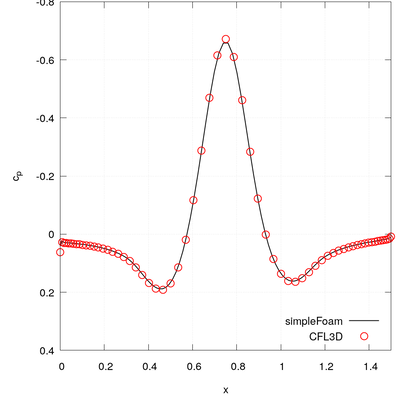## Overview🔗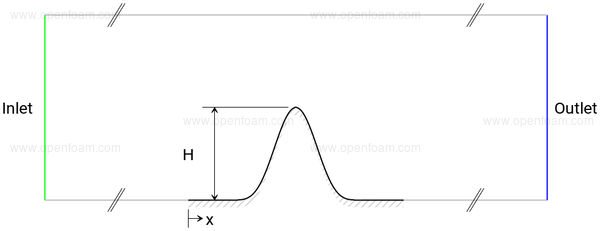## Mesh🔗

• 2D structured mesh created using blockMesh
• bump described

$y(x) = \begin{cases} 0.05 [\sin(\pi \frac{x}{0.9}-\frac{\pi}{3})]^4, & 0.3 \le x \le 1.2, \\ 0 , & 0 \le x \lt 0.3 \, \text{and} \, 1.2 \lt x \le 1.5. \end{cases}$
• this has been set in the blockMeshDict using a codeStream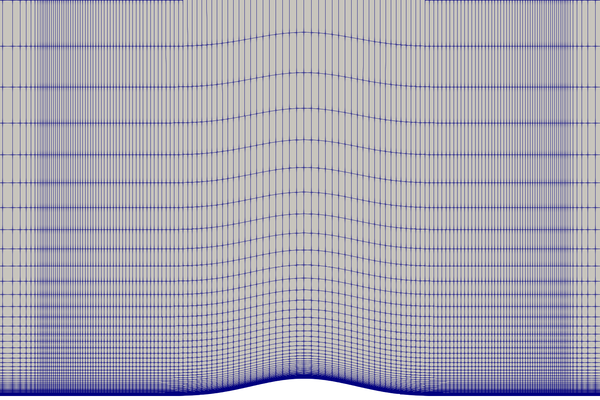## Boundary conditions🔗

• $$U$$ based on a Mach number of 0.2. Mach number, $$Ma$$, is defined as:

$Ma = \sqrt{\gamma R T}$

where $$\gamma$$ is the ratio of specific heats, $$R$$ the gas constant and $$T$$ the temperature. Using values for air at 300K, the inflow velocity is given as:

$U = 0.2 Ma = 0.2 \sqrt{1.4 \times 287 \times 300} = 69.44 m/s$
• The laminar viscosity is derived from the Reynolds number, i.e.

$\nu_\infty = \frac{|\u| L}{Re} = \frac{69.44 \times 1}{3 \times 10^6} = 2.31 \times 10^{-5} m^2/s$

### Common fields🔗

Velocity: U

Patch Condition Value
Inlet fixedValue 69.44 m/s in x
Bump noSlip
Walls symmetryPlane

Pressure: p

Patch Condition Value
Outlet fixedValue 0 Pa (static)
Walls symmetryPlane

### Turbulence fields🔗

Turbulence viscosity: nut

Patch Condition Value
Inlet calculated
Outlet calculated
Bump nutUSpaldingWallFunction
Walls symmetryPlane

#### Spalart-Allmaras

Modified turbulence viscosity: nuTilda

Patch Condition Value
Inlet fixedValue based on $$3 \nu_\infty$$